# KSEEB Solutions for Class 6 Maths Chapter 6 Integers Ex 6.1

Students can Download Chapter 6 Integers Ex 6.1 Questions and Answers, Notes Pdf, KSEEB Solutions for Class 6 Maths helps you to revise the complete Karnataka State Board Syllabus and score more marks in your examinations.

## Karnataka State Syllabus Class 6 Maths Chapter 6 Integers Ex 6.1

Question 1.
Write opposites of the following
Solution:
a) Increase in Weight
Decrease in weight

b) 30 km north
30km south

c) 326 BC
326 A.D

d) Loss of Rs 700
Gain of Rs.700

e) 100m above sea level
100m below sea levelQuestion 2.
Represent the following numbers as integers with appropriate signs
Solution:
a) An aeroplane is flying at a height two thousand metre above the ground
+2000

b) A submarine is moving at a depth, eight hundred metre below the sea level.
– 800

c) A deposit of rupees two hundred
+ 200

d) Withdrawal of rupees seven hundred.
– 700

Question 3.
Represent the following numbers on a number line:
Solution:
a) +5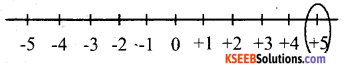b) -10c) +8d) -1e) -6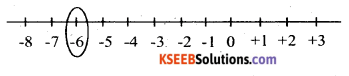Question 4.
Adjacent figure is a vertical number line, representing integers. Observe it and locate the following points:
Solution:
a) If point D is +8, then which point is -8?
-F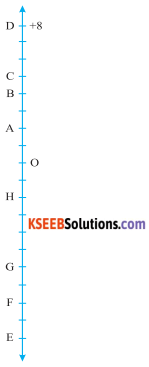b) Is point G a negative integer or a positive integer?
Negative integer (-6)

c) Write integer for points B and E.
Point B represents 4 and point E represents -10

d) Which point marked on this number line has the least value?
E has the least value as it represents -10

e) Arrange all the list of temperatures of five places in India on a particular day of the year.
D > O > B > A > O > H > G > F > E.Question 5.
Following is the list of temperatures of five places in India on a particular day of the year.a) Write the temperatures of these places in the form of integers in the blank column.
b) Following is the number line representing the temperature in degree Celsius.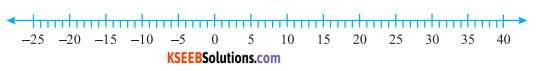Plot the name of the city against its temperature.
c) Which is the coolest place?
d) Write the names of the places where temperatures are above 10°C.
Solution :c) Siachin

Question 6.
In each of the following pairs, Which number is to the right of the other on the number line?
Solution:
a) 2, 9
9(9 > 2)

b) -3, -8
-3 (-3 > -8)

c) 0, -1
0( 0 > -1)

d) -11, 10
10(10 > -11)

e) -6, 6
6(6 > -6)

f) 1, -100
1(1 > -100)Question 7.
Write all the integers between the given pairs ( Write them in the increasing order)
Solution:
a) 0 and -7
-6, -5, -4, -3, -2, -1

b) -4 and 4
-3, -2, -1, 0, 1, 2, 3

c) -8 and -15
-14, -13, -12, -11, -10, -9

d) -30 and -23 .
-29, -28, -27, -26, -25, -24

Question 8.
a)Write four negative integers greater than -20
Solution:
-19, -18, -17, -16

b) Write four negative integers less than -10
Solution:
– 11, – 12, – 13, – 14

Question 9.
For the following statements, Write True(T) or False(F). If the statement is false, correct the statement
Solution:
a) -8 is to the right of -10 on a number line
True (-8 > -10)

b) -100 is to the right of -50 on a number line.
False (-50 > -100)
-100 is to the left of -50 on a number line.

c) smallest negative integer is -1
False as the greater negative integer is -1

d) – 26 is greater than – 25
False as -26 is smallest than -25Question 10.
Draw a number line and answer the following:
Solution:
a) Which number will we reach if we move 4 numbers to the right of -2.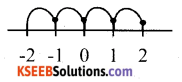We will reach at 2 if we move 4 numbers to the right of -2

b) Which number will we reach if we move 5 numbers to the left of 1.We will reach at -4, if we move 5 numbers to the left of 1.

c) If we are at -8 on the number line, in which direction should we move to reach -1?Clearly, -1 is to the right of -8 Therefore, we should move towards the right direction.

d) If we are at -6 on the number line, in Which direction should we move to reach -1?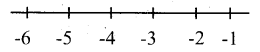Clearly, -1 is to the right of -6. Therefore, we should move towards the right direction.

error: Content is protected !!